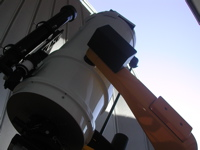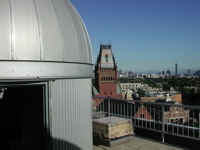# Astronomy Lab and Clay TelescopeMeasure Az, El coordinates of Altair and Mizar

to measure Seasonal motion of the stars

SPU-21 Fall 2015

printer version

This lab should serve as an introduction to the night sky. Positions of celestial objects on the sky are measured with two angles: i) azimuth, or the horizontal (in the plane of the ground) angle of the direction to an object vs. the direction to the North, and measured East of North; and ii) elevation, the angle between the object on the sky and the horizon directly below. Celestial positions are changing with time and so three measurements must be made: azimuth, elevation (azimuth and elevation are both in degrees) and time (date and time, to the nearest 30 seconds).

We will measure one star, Altair, in the Summer Triangle and another star, Mizar, in the handle of the Big Dipper on the Science Center roof. The Summer Triangle is a summer asterism and is composed of three very bright stars, Vega (in the constellation Lyra), Deneb (in the constellation Cygnus, the swan) and Altair (in the constellation Aquila, the eagle), so it will be easy to locate.

There will be 2 small Meade telescopes on the roof of the Science Center pointing at the above 2 stars. These telescopes have been aligned to the North Star, Polaris, so the azimuth angle is the number of degrees from True North. Azimuth angles can then range from 0 degrees (North), to 90 degrees (East), to 180 degrees (South), to 270 degrees (West) and then back to 360 degrees (North). Elevation, also referred to as altitude, angles will range from 0 degrees (on the horizon) to 90 degrees (on the zenith).

What you need:

• a red flashlight
• paper and pencil
• clock

Procedure:

• You should first visually locate the Summer Triangle and the Big Dipper in the night sky. Your TF will help you locate the asterism. Your TF will help you locate these stars. Here's a finder chart of the Altair and Mizar to help!
• We will measure the Az, El coordinates of Altair and then Mizar at least once (each) in Week 1) and then again at as close as possible to the same local time (hour and minute) in Week 2. Our small telescopes are not tracking the star so before we take measurements we need to make sure they are aligned in the reticle eyepiece. One person should record the elevation angle from the side knob of the telescope and the azimuth angle from the bottom of the telescope, while the other person records the accurate time off the clock.
• Notice that there are 24 numbers on the bottom (azimuth) dial. These refer to the hour angle (24 hours in one day). Since there are 360 degrees of azimuth, each number is 15 degrees. Use the fine divisions to measure azimuth to within (at least) 5 degrees.
• Every person in your group should record their own data. This means that every person should make a measurement and then estimate an uncertainty. You will then have 2-3 measurements per group if you pool your data at the end of the session.
• Be sure to take a measurement at the start of lab and at the end of lab. Try to take measurements as close to the same time every night, if possible.

Analysis:

For each star, please pair up your data from the two weeks (one measurement from the first week paired with one from the second week) such that the measurements are done at the same time (closest in hour and minutes). If you were not able to take the measurements at the same time, pair the measurements such that they are as close as possible.

• Fill in all the columns that are marked in green. All the other columns will be automatically filled out for you.
• For the latitude, use 42.38 (enter in degrees; the Excel doc will convert to radians for you)
• For the declination of the stars (also enter in degrees) use:
Altair 8.86832222
Mizar 54.9253528
• The red columns are the results of the calculations described in this document. They give the apparent movement of the stars in degrees, during the time interval between the two measurements. There are two methods by which one can get the result, which are equivalent but have a different dependence on the original data which can lead to different errors. The document explains the calculations. We ask that you look at Fig. 1 and try to understand (or better visualize) what we are trying to measure: the angle "x" of rotation (between your 2 observations S1 and S2) about the North Celestial Pole. The 2 Methods get at this angle by 2 different triangles (drawn in red, in Figs. 4 and 5) that each have the common side, S1 to S2. So you don't need to reproduce or copy down any of the math; this is just help you visualize how the analysis can be done (by either method) by applying the cosine rule to these triangles on the sphere of the sky seen by the observer (you). So include a sentence or two to relate your understanding of this.